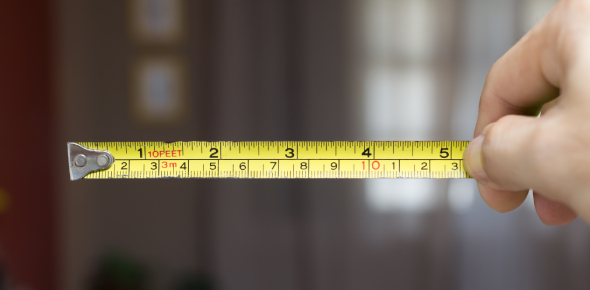# The Metric System MCQ Exam: Trivia!

20 Questions | Total Attempts: 113Settings.

• 1.
1.5 km = _______ m
• A.

.015

• B.

.00015

• C.

150

• D.

1500

• 2.
6 m = ___________cm
• A.

6

• B.

600

• C.

.06

• D.

.006

• 3.
333 cm = 3.33
• A.

Dm

• B.

Km

• C.

M

• D.

Mm

• 4.
1 m = ___________
• A.

100 cm

• B.

1000 cm

• C.

.001 cm

• 5.
The metric system is based upon what number?
• A.

5

• B.

3

• C.

10

• D.

25

• 6.
"Kilo" means...
• A.

1000x

• B.

100x

• C.

10x

• D.

5x

• 7.
"Centi" means...
• A.

100x

• B.

1/10

• C.

1/100

• D.

10x

• 8.
What is the metric unit for measuring length?
• A.

Inch

• B.

Meter

• C.

Foot

• D.

Yard

• 9.
2000mL = ____________L
• 10.
500kL = ____________hL
• 11.
500km = ____________hm
• 12.
25600mL= ________________L
• 13.
________kL = 3400L
• 14.
1,500cg  = _____________150kg
• A.

True

• B.

False

• 15.
15cm = 1.5mm
• A.

True

• B.

False

• 16.
6250mL= _____________L
• 17.
The smartest boy was born on 12/12/12. His brain size was estimated to be 200kg, his brain size in grams is
• 18.
145 mm = ________m
• A.

.0145

• B.

1.45

• C.

145,000

• D.

0.145

• 19.
What is the metric unit for measuring mass or weight?
• A.

Foot

• B.

Pound

• C.

Kilogram

• D.

Ounce

• 20.
5mL = _________L
Related TopicsBack to top